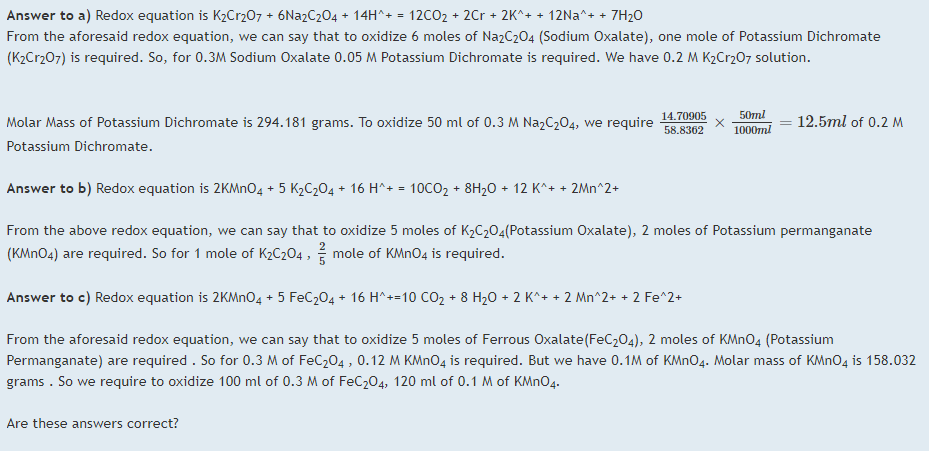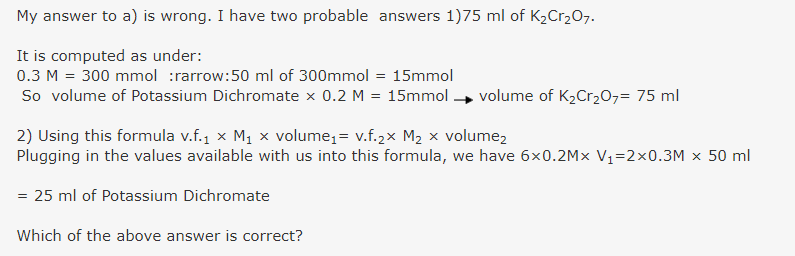# Equivalent based questions (valency factors)

## Recommended Posts

a) What volume of 0.2 M K2Cr2O7 is required to oxidize 50 ml of 0.3 M Na2C2O4 in acidic medium?

b)How many moles of KMnO4 will be required to react completely with 1 mole of K2C2O4 in acidic medium?

c) What volume of 0.1 M KMnO4 is required to oxidize 100 ml of 0.3M FeC2O4 (Ferrous Oxalate) in acidic medium?

d) How many moles of FeC2O4 is required to reduce 2 mol of KMnO4 in acidic medium?

e)How many moles of K2Cr2O7 are required to react completely with 2.5 moles of Cu2S in acidic medium?

My attempt to answer all these questions:

a) 75 mL of 0.2M K2Cr2O7 is required to oxidize 50 mL of 03M Na2C2O4 in acidic medium.

b) 0.4 mole of KMnO4 is required to react completely with 1 mole of K2C2O4 in acidic medium.

c) 120 mL of 0.1M KMnO4 is required to oxidize 100 mL of 0.3M FeC2O4 in acidic medium.

d) 5 moles of FeC2O4 are required to reduce 2 moles of KMnO4 in acidic medium.

e) 5 moles of K2Cr2O7 are required to react completely with 2.5 moles of Cu2S in acidic medium.

Are these above answers correct ?
Let me know. Thanks.

##### Share on other sites

Are the following rectified answers to a, b, c correct?##### Share on other sites##### Share on other sites

22 hours ago, Dhamnekar Win,odd said:

Hello,

Both of these above answers are wrong. So ignore the contents of this post.

##### Share on other sites

Answer to d: 5 moles of Ferrous oxalate FeC2O4  are required to reduce 2 moles of Potassium Permanganate in acidic medium.

Redox equation is  $K_2Cr_2O_7 + 3Cu_2S + 14H^+ \rightarrow 6Cu^{2+} + 3S + 7 H_2O + 2K^+ + 2Cr$
From the aforesaid equation, we can say that $\frac56$ moles of   K2Cr2O7 is required to react completely with 2.5 moles of  $Cu_2S$ in acidic medium.

Edited by Dhamnekar Win,odd

## Create an account

Register a new account